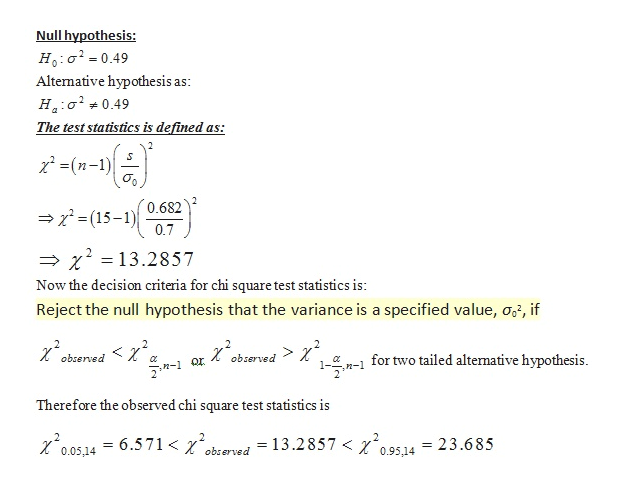# Use a χ2​-test to test the claim σ2=0.49 at the α=0.10 significance level using sample statistics s2=0.465 and n=15.Assume the population is normally distributed.

Question
9 views

Use a χ2​-test to test the claim σ2=0.49 at the α=0.10 significance level using sample statistics s2=0.465 and n=15.

Assume the population is normally distributed.

check_circle

Step 1

In order to test the hypothesis of H0: sigma square  is equal to 0.49  versus Ha: sigma square not equal to 0.49 using a  10% level of significance with a random sample from a normal population shows that the sample size n is equal to 15 and standard deviation is equal to 0.682.

We define the test hypothesis as:

Null hypothesis as: H0: sigma square is equal to 0.49

Against the alternative hypothesis as;

Ha: sigma square not equal to 0.49

In order to test this hypothesis we used chi square test statistics as follows:

Step 2

In order to test this hypothesis we used chi square test statistics as follows:

Test Statistics:

The chi- square hypothesis test is defined as;

Null hypothesis:help_outlineImage TranscriptioncloseNull hypothesis: Ho a 0.49 Altemative hypothe sis as: Ha 0.49 The test statistics is defined as: # S (n-) 0.682 (15-1) 0.7 2 = 13.2857 Now the decision criteria for chi square test statistics is: Reject the null hypothesis that the variance is a specified value, o, if 2 > x 1H- for two tailed altemative hypothesis obrerved observed Therefore the observed chi square test statistics is =13.2857 < % 0.95,14 o05 14=6.571< gbserved 23.685 fullscreen
Step 3

P value for this test is deter...

### Want to see the full answer?

See Solution

#### Want to see this answer and more?

Solutions are written by subject experts who are available 24/7. Questions are typically answered within 1 hour.*

See Solution
*Response times may vary by subject and question.
Tagged in

### Statistics